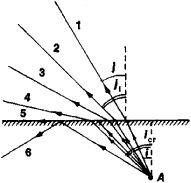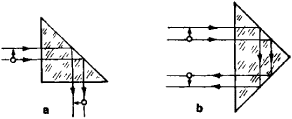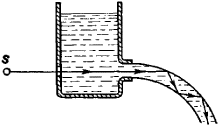# total internal reflection

(redirected from Frustrated total internal reflection)
Also found in: Dictionary, Acronyms.

## total internal reflection

[′tōd·əl in′tərn·əl ri′flek·shən]
(optics)
A phenomenon in which electromagnetic radiation in a given medium which is incident on the boundary with a less-dense medium (one having a lower index of refraction) at an angle less than the critical angle is completely reflected from the boundary.

## Total Internal Reflection

When electromagnetic radiation, such as light or radiowaves, in a transparent medium with a higher refractive index meets the boundary of a transparent medium with a lower refractive index at an angle of incidence i greater than a certain critical angle iCr, total internal reflection occurs. When i > icr, refraction into the second medium ceases.Figure 1. Illustration of total internal reflection: (A) light source. Total internal reflection (ray 6) occurs when the angles of incidence of light on the surface of the optically less dense medium exceed the critical angle icr for which the angle of refraction i is 90° (ray 5). The refractive index of the lower medium is greater than that of the upper medium.

Total internal reflection was first described by J. Kepler. After the discovery of Snell’s law of refraction, it became clear that within the framework of geometrical optics total internal reflection follows directly from this law, since the angle of refraction j cannot exceed 90° (Figure 1). The quantity icr is given by the condition sin icr = 1/n, where n is the relative refractive index of the first and second media. Because of dispersion, the values of n and, consequently, of icr differ somewhat for different wavelengths (frequencies) of radiation.Figure 2. Total-reflecting prisms: (a) deviating prism, (b) reversing prism; the arrows on the lines representing light rays indicate the direction of the rays

In total internal reflection the electromagnetic energy is completely returned— hence the term “total”—to the optically denser medium, that is, the medium with the higher refractive index. The reflection coefficient in total internal reflection is greater than in specular reflection from polished surfaces and is practically equal to unity. Moreover, for total internal reflection, unlike specular reflection, the coefficient is independent of the wavelength of the radiation, provided that total internal reflection occurs for the wavelength; even in the case of repeated total internal reflection the spectral composition (“color”) of complex radiation does not change. For this reason, extensive use is madeFigure 3. Total internal reflection in a liquid jet: (S) light source

of total internal reflection in many optical instruments and experiments (see Figures 2 and 3). It should be noted, however, that the energy of electromagnetic waves during total internal reflection partially penetrates into the second medium (the medium with the lower refractive index) but then returns. The depth of this penetration is extremely slight and is of the order of the wavelength of the reflected light.

### REFERENCE

Landsberg, G. S. Optika, 4th ed. Moscow, 1957. (Obshchii kurs fiziki, vol. 3.)
Born, M., and E. Wolf. Osnovy optiki, 2nd ed. Moscow, 1973. (Translated from English.)
Tolansky, S. Udivitel’nye svoistva sveta. Moscow, 1969. (Translated from English.)

## total internal reflection

Light that is reflected back from the edge of the medium it is traveling through. When light rays travel at an angle greater than the "critical" angle, which is determined by the medium, the light reflects back into the medium. When they are less than the critical angle (more perpendicular), the light is refracted out of the medium and lost to the outside. See refractive index.
Site: Follow: Share:
Open / Close